# Quiz 13: The Balanced Scorecard: Strategic-Based Control

Cycle time: Cycle time is the time it takes to produce a unit of output from the time the materials are received till the finished goods are produces. The data of the production department of the company is given in the question. Given:1.The actual cycle time is calculated as:The standard cost per unit is calculated asHence, the actual conversion cost per unit is calculated as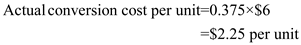Hence, the actual conversion cost per unit is 2.The theoretical cycle time is calculated as:The standard cost per unit is calculated asHence, the actual conversion cost per unit is calculated as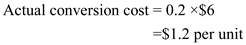Hence, the ideal conversion cost per unit is 3.If the actual velocity is 220 units per hour then the actual cycle time is calculated as:Hence, the actual conversion cost per unit is calculated as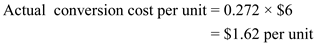This would not make much difference in the delivery and performance.

A strategic-based responsibility accounting system is a system which is responsible for modifying the corporation's policy and strategy with operational goals and planning for four perspectives, which are as under: 1. The process perspective, 2. The learning and growth perspective, 3. The financial perspective 4. The customer perspective The differentiation between strategic-based responsibility accounting system and activity-based responsibility accounting system is that strategic-based responsibility accounting system has the legal connections with strategy and it includes two of perspectives in the responsibility structure: The learning and growth perspective and the customer perspective however, activity-based responsibility accounting system does not have any legal connections with strategy and it does not include any of the four perspectives in the responsibility structure.

Manufacturing cycle efficiency: MCE (manufacturing cycle efficiency) is another time-based operational measure. The information of one of its products for each hour of production is given in the question. Given information:1.Process time is calculated as: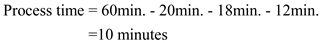The MCE is calculated as:Hence, the MCE is The value of 0.17 indicates that 83% of the manufacturing cycle time plus the waste. 2.Theoretical cycle time is calculated below:The actual cycle time is calculated as:Hence, the MCE is calculated asThe MCE is 3.New waste is calculated below: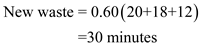Hence, the MCE is calculated asThe MCE is

There is no answer for this question

There is no answer for this question

There is no answer for this question

There is no answer for this question

There is no answer for this question

There is no answer for this question